# RLC Parallel Circuit

Finding the impedance of a parallel RLC circuit is considerably more difficult than finding the series RLC impedance. This is because each branch has a phase angle and they cannot be combined in a simple way. The impedance of the parallel branches combine in the same way that parallel resistors combine: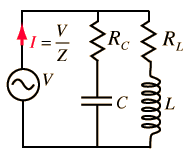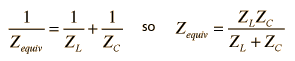But although the branch impedance magnitudes can be calculated from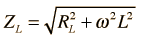and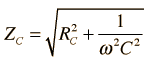RL Circuit
 RC Circuit
they cannot be directly combined as suggested by the expression above because they are different in phase - like vectors in different directions cannot be added directly. This dilemma is most easily solved by the complex impedance method.

Index

AC Circuits

 HyperPhysics***** Electricity and Magnetism R Nave
Go Back

# RLC Parallel: Complex Impedance Method

When the complex impedances of the branches of the parallel RLC circuit are combined, the equivalent impedance is of the form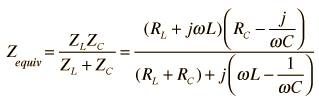When this expression is rationalized and put in the standard form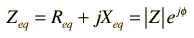Calculation

then the impedance in ohms and the phase can be determined. By setting the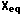= 0, the resonant frequency can be calculated. The expressions for these calculations are quite lengthy.

Index

AC Circuits

 HyperPhysics***** Electricity and Magnetism R Nave
Go Back

# RLC Parallel Expressions

The complex impedances of the parallel RLC circuit takes the form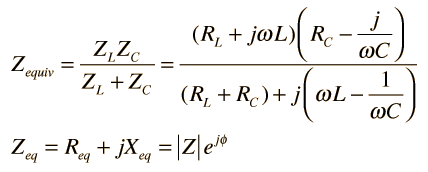when rationalized, and the components have the form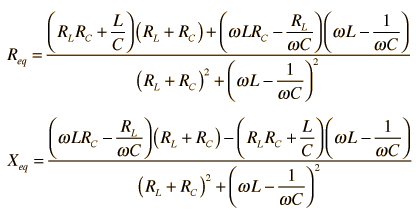Calculation
Index

AC Circuits

 HyperPhysics***** Electricity and Magnetism R Nave
Go Back